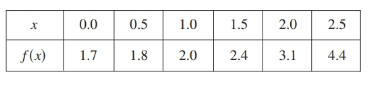# Find the value of g ' ( 1 ) using given relation and table.### Single Variable Calculus: Concepts...

4th Edition
James Stewart
Publisher: Cengage Learning
ISBN: 9781337687805### Single Variable Calculus: Concepts...

4th Edition
James Stewart
Publisher: Cengage Learning
ISBN: 9781337687805

#### Solutions

Chapter 3.4, Problem 58E
To determine

## Find the value of g'(1) using given relation and table.

Expert Solution

The value of the given expression g'(1)=1.2 .

### Explanation of Solution

Given:

The given relation is g(x)=f(f(x)) and the table is given below.Calculation:

Use formula g'(x)=(f(f(x)))'=f'(f(x))f'(x)

f(1)=2f'(1)=2.41.81.50.5=0.61=0.6f'(f(1))=f'(2)=4.42.42.51.5=21=2

Now

g'(1)=(f(f(1)))'=f'(f(1))f'(1)g'(1)=(f(f(1)))'=2(0.6)=1.2

Hence thevalue of the given expression g'(1)=1.2 .

### Have a homework question?

Subscribe to bartleby learn! Ask subject matter experts 30 homework questions each month. Plus, you’ll have access to millions of step-by-step textbook answers!